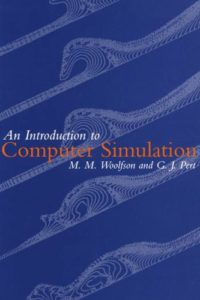اسم المؤلف
M. M. Woolfson, G. J. Pert
التاريخ
3 أبريل 2018
المشاهدات
التقييم(لا توجد تقييمات)Loading...

An Introduction to Computer Simulation
M. M. Woolfson, G. J. Pert
Department of Physics,
University of York
Contents
List of programs available xv
1 Models and simulation l
1.1 What is a model? 1
1.2 Why we use models and how 3
1.2.1 A simple harmonic oscillator 3
1.3 Techniques for solving first-order ordinary 6
differential equations
1.3.1 Euler methods 6
1.3.2 The Runge-Kutta method 8
1.3.4 The Numerov method 11
1.4 Solution of the forced, damped oscillator 12
1.5 General ideas about simulation 14
1.5.1 The Trojan asteroids 15
1.5.2 An orbiting satellite under tidal stress 17
1.6 Types of simulation and their applications 22
1.6.1 The Monte Carlo method 22
1.6.2 Particle methods 24
1.6.3 Continuum physics – the finite-difference
method 26
1.6.4 Continuum physics – the finite-element method 27
1.7 Complex models – a systems approach 28
1.8 Validation and testing 30
Problems 31
Finite-difference methods 33
2.1 Finite differences for ordinary derivatives 33
2.2 The use of finite differences 37
2.2.1 The shooting method 38
2.2.2 Solution by linear equations 39
2.3 The diffusion equation 41
2Contents
2.4 Finite-difference representation of partial derivatives 43
2.5 The explicit method 44
2.6 The Crank-Nicholson implicit method 47
2.7 Differential boundary conditions 50
2.8 The Dufort-Frankel method 53
2.9 Consistency 55
2.9.1 Consistency for the explicit method 57
2.9.2 Consistency for the Crank-Nicholson
method 59
2.10 Stability 61
2.10.1 Stability of the explicit method 61
2.10.2 Stability of the Crank-Nicholson
method 63
2.11 Types of partial differential equation 64
2.12 A heated plate in equilibrium 64
methods
2.13.1 The Gauss-Seidel method
2.13.2 The relaxation method
2.13.3 The over-relaxation method
2.14 The solution of general heated-plate problems
2.15 The Poisson, Laplace and diffusion equations
with other geometries
2.15.1 The control-volume method
2.15.2 A heat-flow problem with axial
symmetry
Problems
Simulation with particles
3.1 Types of problem
3.2 A few particles with inter-particle forces
3.2.1 A binary-star system
3.3 An electron in a magnetic field
3.3.1 The electron-microscope lens
3.4 N-body problems
3.4.1 Evolution of a stellar cluster
3.5 Molecular dynamics
3.5.1 Simulation of a liquid by molecular dynamics
3Contents xi
3.5.2 The equation of state of a liquid 102
3.5.3 The equation of state from molecular
dynamics 104
3.6 Considerations in modelling plasmas 108
3.7 The collisionless particle-in-cell model 114
3.7.1 A one-dimensional plasma program 116
3.8 Galaxy simulation 122
3.9 Fourier-series methods of solving Poisson’s
equation 125
Problems 127
The Monte Carlo method 129
4.1 Applications of Monte Carlo simulations 129
4.2 Thegeneration of random numbers – uniform
deviates 130
4.2.1 Sequential correlation 130
4.2.2 The selection of random points within
a sphere 131
4.3 The generation of random numbers – non-uniform
deviates 132
4.3.1 The transformation method 132
4.3.2 The normal distribution – the Box-Muller
transformation 134
4.3.3 The rejection method 135
4.3.4 Markov chain methods 137
4.4 Simulation of a fluid by a Monte Carlo method 138
R TheMetropolis algorithm – theequation
of state of a liquid 139
4.5 Integration by Monte Carlo methods 141
4.6 Monte Carlo solution of Poisson’s equation 145
4.7 The Monte Carlo method applied to a nuclear
reactor 147
Problems 153
The wave equation 156
5.1 General solutions of the wave equation 156
5.2 A finite-difference approach 158
5.2.1 Stability of the finite-difference equations 160
5.3 Two-dimensional vibrations 164
4 5Contents
5.4 Optical diffraction 166
5.5 Fraunhofer diffraction 168
5.6 Discrete Fourier transforms 169
5.6.1 Fast Fourier transforms 170
5.7 Fresnel diffraction 175
Problems 177
The finite-element method 179
6.1 Introduction 179
6.2 Differential equations and functionals 179
6.3 Linear variational functions 183
6.3.1 Many-straight-line variational
functions 185
6.4 Applications of the finite-element method
in one dimension 190
6.4.1 Time-dependent heat flow 190
6.4.2 Heat flow with differential boundary
conditions 191
6.4.3 Heat generation, point sources and sinks 193
6.4.4 A one-dimensional finite-elementprogram 194
6.5 The general finite-element approach 196
6.6 Laplace’s equation in a two-dimensional space 197
6.6.1 The time-independent heated-plate problem 200
6.7 A two-dimensional finite-element program 202
6.8 Applications of the finite-element method 204
6.9 The residual formulation of finite-element methods 205
6.9.1 Laplace’s equations with the Galerkin method 207
Problems 208
Computational fluid dynamics 211
7.1 A general description of fluid dynamics 211
7.2 The equations of transport of fluid properties 212
7.3 Numerical studies of diffusion and advection 213
7.4 Stability conditions 218
7.5 Central difference for advection and diffusion 221
7.6 The Lax-Wendroff method 223
7.7 The QUICKEST method 225
6 7Contents xiii
7.8 The basic equations of fluid dynamics 227
7.8.1 Conservation of mass – Eulerian form 228
7.8.2 Conservation of momentum –
Eulerian form 229
7.8.3 Conservation of mass and momentum –
Lagrangian form 230
7.9 The incompressible fluid 232
7.10 The pressure method for incompressible fluids 233
7.11 The marker-and-cell method 237
7.12 Polytropic gases 240
7.13 The von Neumann-Richtmyer method for
compressible fluids 243
7.14 Artificial viscosity 245
7.15 Blast-wave modelling 247
7.15.1 A nuclear explosion program 248
7.16 Multi-dimensional compressible flow 250
7.17 Smoothed particle hydrodynamics 251
Problems 255
Appendices
1 The elements of matrix algebra 259
2 A simple conjugate gradient method 265
3 The virial theorem 268
4 The condition for collisionless PIC 270
5 The coefficients of a half-sine-wave Fourier series 273
7 Calculation of the four-element solution to (6.11) 284
Problems – solutions and comments 287
References 307
Index 30
كلمة سر فك الضغط : books-world.net